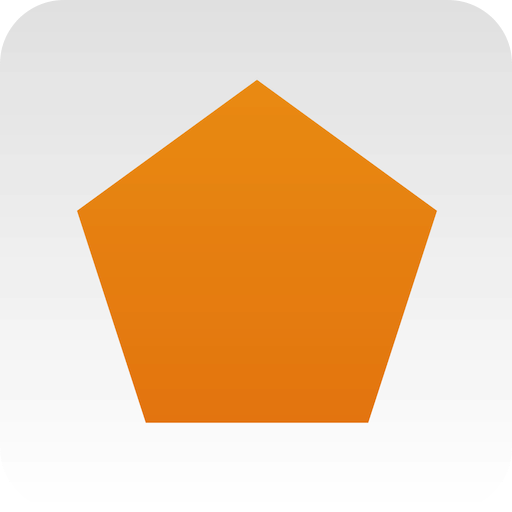# Geometrics – OverviewGeometrics is a powerful productivity and educational tool for the professional or student.

Geometrics is a shape solver. After you provide the right combination of dimensions for a shape, the app determines the rest and then calculates the area and perimeter. Geometrics supports these common two-dimensional shapes:

• Circular: circle, ellipse, sector, segment, ring sector
• Triangles: right, equilateral, isosceles, acute, obtuse
• Quads: square, rectangle, isosceles trapezoid, parallelogram, rhombus
• Polygons: triangle to icosagon (20-sided)

Circle circumference and area is shown numerically or in terms of π. Special 30-60-90 and 45-45-90 triangles can be constructed with areas and perimeters calculated in terms of √3 or √2. Hexagon perimeter and area is shown numerically or in terms of √3.

Geometrics becomes a powerful productivity tool by managing a group of saved shapes. With a simple tap, the app remembers the shape just solved and then sums the saved shape areas to provide a total area. Saved shapes can be viewed and edited at any time. Each saved shape can be scaled to a fraction of its original area, and even can be subtracted from the total area. These features allow Geometrics to construct any arbitrary shape, including those with a “punch out”.

If there is a need to calculate the area of an irregularly shaped concrete patio with a tree growing in the middle of it, let Geometrics do the hard work. To figure out how much area needs to be covered by mulch in intricate landscaping, Geometrics is up to the task! To know how big of an area will be painted in a great room with complex, vaulted ceilings, look no further than Geometrics to provide the answer.

Once saved shapes are edited and arranged to calculate the proper total area, Geometrics can e-mail a report of the saved shapes and the total area. The e-mail contains a table that makes it easy to copy/paste the data into a spreadsheet program.

Watch the video demonstration!